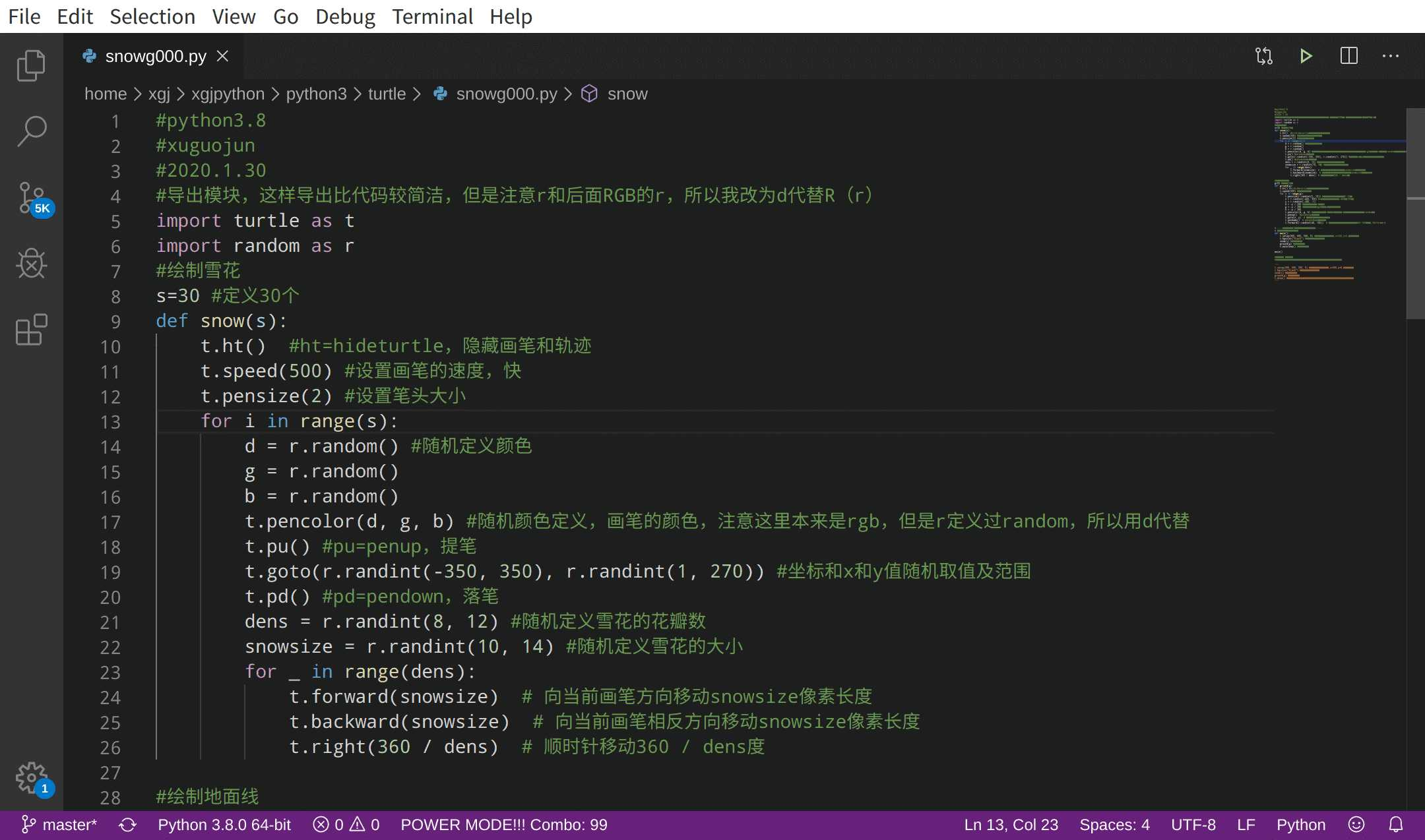# python-turtle-画雪花-2种方法及效果的详解

1.方法一：```#python3.8
#xuguojun
#2020.1.30
#导出模块
import turtle as t
import random as r
#定义画雪
def drawsnow():
t.ht()  #隐藏笔头，ht=hideturtle
t.pensize(2)  #定义笔头大小
for i in range(50): #50，其实画49朵雪花
t.pencolor("white") #定义画笔颜色为白色，其实就是雪花为白色
t.pu() #提笔，pu=penup
t.setx(r.randint(-350,350)) #定义x坐标，随机从-350到350之间选择
t.sety(r.randint(1,350)) #定义y坐标，注意雪花一般在地上不会落下，所以定义是从1开始
t.pd() #落笔，pd=pendown
dens = 6 #雪花瓣数设为6
snowsize = r.randint(2,12) #定义雪花大小
for j in range(dens): #就是6，那就是画5次，也就是一个雪花五角星
#t.forward(int(snowsize))  #int（）取整数
t.fd(int(snowsize))
t.backward(int(snowsize))
#t.bd(int(snowsize))  #注意没有bd=backward，但有fd=forward，小bug
t.right(int(360/dens))  #转动角度

#画地面线：
def drawgroud():
t.ht() #隐藏画笔，t.hideturtle()
for i in range(r.randint(10,15)): #随机画几条地面线，但在10和15之间
#for i in range(10): #每次操作只画10条地面线
x=r.randint(-400,350)
y=r.randint(-280,-1)
t.pencolor("white")
t.pu() #提笔pu=penup
t.goto(x,y) #去这个坐标
t.pd() #落笔pd=pendown
t.fd(r.randint(40,100))  #fd=forward向前画大小，随机从40~100选

t.setup(800,600,200,200)#窗口大小和位置
t.tracer(True)   #雪花和背景绘制的过程,true=就是慢慢看它画雪
t.bgcolor("lightpink") #lightpink=轻粉色，天蓝色lightblue=#B0E0E6
t.speed(0.1) #画笔的速度
drawsnow() #执行画雪
drawgroud() #执行画地面线
t.done() #完成```
View Code2.方法二：```#python3.8
#xuguojun
#2020.1.30
#导出模块，这样导出比代码较简洁，但是注意r和后面RGB的r，所以我改为d代替R（r）
import turtle as t
import random as r
#绘制雪花
s=30 #定义30个
def snow(s):
t.ht()  #ht=hideturtle，隐藏画笔和轨迹
t.speed(500) #设置画笔的速度，快
t.pensize(2) #设置笔头大小
for i in range(s):
d = r.random() #随机定义颜色
g = r.random()
b = r.random()
t.pencolor(d, g, b) #随机颜色定义，画笔的颜色，注意这里本来是rgb，但是r定义过random，所以用d代替
t.pu() #pu=penup，提笔
t.goto(r.randint(-350, 350), r.randint(1, 270)) #坐标和x和y值随机取值及范围
t.pd() #pd=pendown，落笔
dens = r.randint(8, 12) #随机定义雪花的花瓣数
snowsize = r.randint(10, 14) #随机定义雪花的大小
for _ in range(dens):
t.forward(snowsize)  # 向当前画笔方向移动snowsize像素长度
t.backward(snowsize)  # 向当前画笔相反方向移动snowsize像素长度
t.right(360 / dens)  # 顺时针移动360 / dens度

#绘制地面线
g=50 #定义50条
def ground(g):
t.ht() #ht=hideturtle，隐藏画笔和轨迹
t.speed(500) #画笔的速度
for i in range(g):
t.pensize(r.randint(5, 10)) #随机画笔大小，从5~10选
x = r.randint(-400, 350) #x坐标轴的随机从-400到350选
y = r.randint(-280, -1)
d = -y / 280 #注意这里的r要该d
g = -y / 280 #定义随机数dgb，与y随机数挂钩
b = -y / 280
t.pencolor(d, g, b) #定义颜色，r改为d，因为r在模块导出时定义random的
t.penup()  #pu=penup，提笔
t.goto(x, y)  # 让画笔移动到此位置
t.pendown()  # pd=pendown，落笔
t.forward(r.randint(40, 100))  # 眼当前画笔方向向前移动40~100距离,fd=forward

#-----熟悉以下2中方法，启动画画------
# 方法一：主函数法
def main():
t.setup(800, 600, 500, 0) #窗口大小和位置,x=500,y=0,代表坐标
t.bgcolor("black") #背景颜色为黑色
snow(s) #代表数量
ground(g) #代表数量
t.mainloop() #循环挂起

main()

#方法二 简洁法
#将方法一，注释掉，去掉下面的注释，启动方法二，即可

‘‘‘
t.setup(800, 600, 500, 0) #窗口大小和位置,x=500,y=0,代表坐标
t.bgcolor("black") #背景颜色为黑色
snow(s) #代表数量
ground(g) #代表数量
t.done() #如果没有这个，结束后自动关闭窗口，有了，就不关闭。
‘‘‘```
View Codepython-turtle-画雪花-2种方法及效果的详解

(0)
(0)